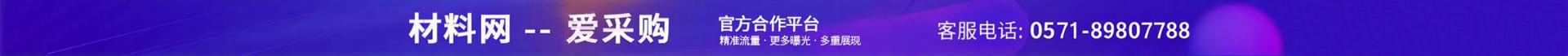•••e="3286" et="1">

me="68539">

e="1634" et="2">

e="932" et="3">

e="889" et="5">